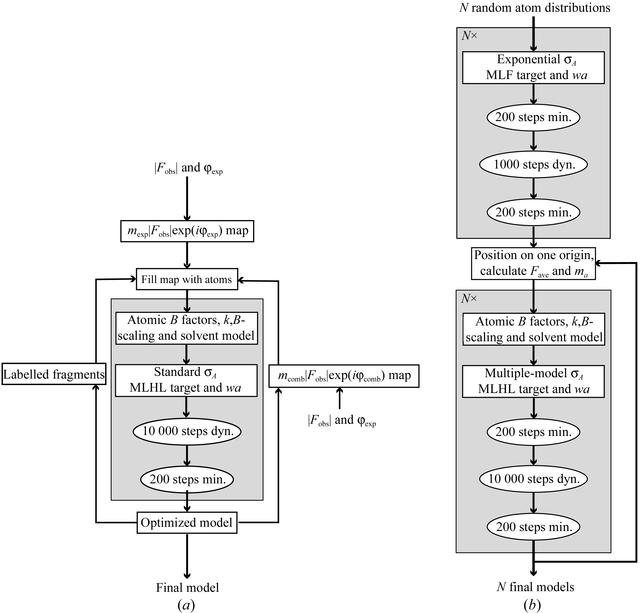disable zoom     view article Figure 1 Protocols for automated model building and ab initio modelling. (a) Automated model building by Conditional Optimization using the program CNS (Brünger et al., 1998). The standard geometrical target functions were replaced by our conditional formulation (see main text). Electron-density maps were filled with loose atoms (as described in the main text). Refinement cycles used the MLHL crystallographic target function and consisted of 10 000 steps of dynamics (denoted `dyn.') and 200 steps energy minimization (`min.'). Velocities in the dynamics calculations were scaled to a constant temperature of 600 K. Overall anisotropic B-factor scaling, bulk-solvent correction, σA estimation, determination of wa weight and phase combination were performed using standard CNS routines. After each cycle, the positions of atoms that could be assigned to protein fragments (see main text) were maintained in the model. Placing the remaining number of atoms in the phase-combined electron-density map completed the starting model for a next cycle of optimization. (b) Multiple-model optimization protocol starting from N models of randomly distributed atoms. The CNS program was used with conditional formulation for the geometrical target functions. First, a short condensation step was performed for each model with 200 steps of minimization and 1000 steps of dynamics followed by 200 steps minimization using the MLF crystallographic target function. Subsequent optimization cycles consisted of 200 steps of minimization, 10 000 steps of dynamics and 200 steps of minimization using the MLHL crystallographic target function. Phases of the averaged structure factors and figures of merit (ma) were used in the phase restraint (see main text). The phased-translation function was used to put the N models on a common origin prior to averaging. The bulk-solvent model was generated for 20%(v/v); atoms present in solvent regions were given zero occupancy.BIOLOGICALCRYSTALLOGRAPHY
ISSN: 1399-0047
Volume 60| Part 12| November 2004| Pages 2202-2209